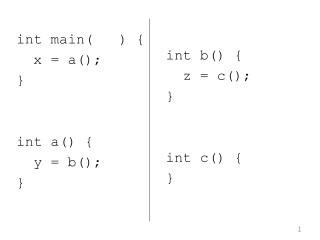DownloadDownload Presentationint main( ) { x = a(); } int a() { y = b(); }

# int main( ) { x = a(); } int a() { y = b(); }

Télécharger la présentation## int main( ) { x = a(); } int a() { y = b(); }

- - - - - - - - - - - - - - - - - - - - - - - - - - - E N D - - - - - - - - - - - - - - - - - - - - - - - - - - -
##### Presentation Transcript

1. int main( ) { x = a(); } int a() { y = b(); } int b() { z = c(); } int c() { }

2. main calls a a calls b b calls c c returns to b b returns to a a returns to main The call tree: main a b c

3. Partial assembly code for main() and a() main: . . branch a # call a_rtn: copy x, a_rtn_value . . a: . . branch b # call b_rtn: copy y, b_rtn_value . . branch a_rtn # return from a

4. Modified assembly code for main() and a() main: . branch a # call a_rtn1: copy x, a_rtn_value . branch a # another call to a a_rtn2: copy x, a_rtn_value . a: . . branch b # call b_rtn: copy y, b_rtn_value . . branch # return from a

5. Different example: Recursion int main( ) { x = a( ); } int a( ) { z = a( ); } main a

6. main: la a_rtn, rtn1 branch a # call rtn1: # copy out return value a: # recursive call la a_rtn, rtn2 branch a rtn2: # copy out return value . . branch (a_rtn)

7. To make this work, the code needs to • just before each call, save the return address • before each return, get and use the most recently saved return address The data structure required is called a stack.

8. A stack organizes data • LIFO – Last In First Out • push puts data into the stack • pop takes data out of the stack

9. a The initial state of the stack: it is empty. After a first push of a onto the stack.

10. c b b a a After a second push of b onto the stack. After a third push of c onto the stack.

11. d b b a a The stack returns c due to a pop operation. Pushd.

12. b a a Pop returns d. Pop returns b.

13. Different example: Recursion main: la a_rtn, rtn1 branch a # call rtn1: # copy out return value a: push a_rtn # recursive call la a_rtn, rtn2 branch a rtn2: # copy out return value . . pop a_rtn branch (a_rtn)

14. The problem of overwritten return addresses is solved with a stack. The same problem exists with • parameters • variables local to a function The solution bundles all these saved/restored values into a single set to be pushed/popped. The set of items is called an activation record (AR) or stack frame.

15. Important detail: A compiler cannot always know if there is recursion in a program! Consider separate compilation. In file 1: int a() { z = b(); } In file 2: int b() { x = a(); }

16. Memory Allocation • What is in memory? • When is it in memory?And, when is or was the memory space allocated? • Where within memory is it?

17. What and Where are • program code (machine code) • program variables (global) • stack • heap Each can be thought of as residing in its own, separate section of memory. These sections are often identified as segments.

18. When is the memory space allocated? • staticThe compiler knows details of the allocation, so causes the assembler to allocate the space. This implies that the memory image created by the assembler contains the memory space. • dynamicThe allocation of memory space occurs while the program executes.

19. Program Code The program's source code is compiled and then it is assembled to produce machine code (memory image). To run the program, the memory image is placed (copied) into memory. Therefore, the code is already in memory, and classified as a static allocation.

20. int x, y, z; /* global variables */ int main() { } Both the compiler, and therefore the assembler, know exactly how much memory space is needed. When the program (memory image) is placed into memory, the allocation of memory for the integers has been completed. It is therefore classified as a static allocation.

21. #define MAX 4 int array[MAX]; /* global */ int main() { }

22. char *stringptr; stringptr = (char *) malloc(bytes_needed + 1);

23. Consider space for the activation record: a: push a_rtn . . la a_rtn, rtn2 branch a rtn2: . . pop a_rtn branch (a_rtn)

24. intfcn(int y) { } int main() { int x, y; y = 20; x = fcn(y); }

25. Summary • Static • program code • global variables • Dynamic • anything that goes onto the stack, such as parameters and return address • memory allocated as the program is running, such as allocation done with malloc()